# Raycasting II: Floor and Ceiling

Back to index

## Introduction

In the previous raycasting article was shown how to render flat untextured walls, and how to render textured ones. The floor and ceiling have always remained flat and untextured however. If you want to keep the floor and ceiling untextured, no extra code is needed, but to have them textured too, more calculations are needed.
Wolfenstein 3D didn't have floor or ceiling textures, but some other raycasting games that followed soon after Wolf3D had them, for example Blake Stone 3D: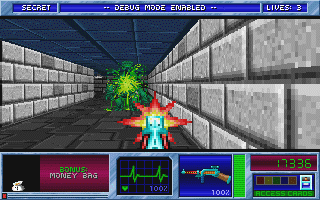You can download the full source code of this tutorial here.

## How it Works

Unlike the wall textures, the floor and ceiling textures are horizontal so they can't be drawn the same way as the wall with vertical stripes. Instead, they're drawn with horizontal scanlines. The perspective is similar to that of walls but 90 degrees rotated, but unlike the walls which used exactly 1 texture per vertical stripes, multiple floor textures (or the same one repeatedly) may cross our horizontal line.

Drawing the ceiling happens the same way as drawing the floor, so only the floor is explained here.

The floor casting is done before the walls, so first we draw the entire floor (and ceiling), then overwrite part of the pixels with the walls, as before, in the next step.
In short, the floor casting works as follows: work scanline by scanline. For the current scanline, compute the position on the floor matching the left pixel of the scanline, and the position matching the right pixel. This can be computed as where the ray starting from the camera, going through that pixel of the camera plane, hits the floor. The formulas and explanation for this are in the floor casting code further below.

We can then linearly interpolate between this leftmost and rightmost point to get the floor coordinates matching the other pi xels of this scanline. This works because the floor texture is perfectly horizontal. If it were slanted, we would need to do more expensive perspective correct texture mapping instead.

NOTE: Ádám Tóth contributed the idea and demo code for the horizontal scanline technique in 2019. Before this, this tutorial described a vertical stripe based technique, but the horizontal technique is faster and matches how raycasting games really worked. The vertical technique is moved to a separate chapter at the end.

## The Code

The code tries to load the wolfenstein textures from the previous raycasting tutorial, you can download them here (copyright by id Software). If you don't want to load textures, you can use the part of code that generates textures from the previous raycasting tutorial instead, but it looks less good.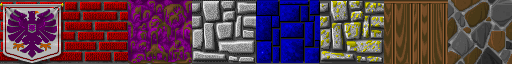The first part of the code is exactly the same as in the previous raycasting tutorial, but is given here to situate where the new code will be. There's also a new map. This piece of code declares all needed variables, loads the textures, and draws textured vertical wall stripes. For the loading of the textures, please see the previous raycasting tutorial about getting the images or using an alternative way to generate the textures.

 ```#define screenWidth 640 #define screenHeight 480 #define texWidth 64 #define texHeight 64 #define mapWidth 24 #define mapHeight 24 int worldMap[mapWidth][mapHeight]= { {8,8,8,8,8,8,8,8,8,8,8,4,4,6,4,4,6,4,6,4,4,4,6,4}, {8,0,0,0,0,0,0,0,0,0,8,4,0,0,0,0,0,0,0,0,0,0,0,4}, {8,0,3,3,0,0,0,0,0,8,8,4,0,0,0,0,0,0,0,0,0,0,0,6}, {8,0,0,3,0,0,0,0,0,0,0,0,0,0,0,0,0,0,0,0,0,0,0,6}, {8,0,3,3,0,0,0,0,0,8,8,4,0,0,0,0,0,0,0,0,0,0,0,4}, {8,0,0,0,0,0,0,0,0,0,8,4,0,0,0,0,0,6,6,6,0,6,4,6}, {8,8,8,8,0,8,8,8,8,8,8,4,4,4,4,4,4,6,0,0,0,0,0,6}, {7,7,7,7,0,7,7,7,7,0,8,0,8,0,8,0,8,4,0,4,0,6,0,6}, {7,7,0,0,0,0,0,0,7,8,0,8,0,8,0,8,8,6,0,0,0,0,0,6}, {7,0,0,0,0,0,0,0,0,0,0,0,0,0,0,0,8,6,0,0,0,0,0,4}, {7,0,0,0,0,0,0,0,0,0,0,0,0,0,0,0,8,6,0,6,0,6,0,6}, {7,7,0,0,0,0,0,0,7,8,0,8,0,8,0,8,8,6,4,6,0,6,6,6}, {7,7,7,7,0,7,7,7,7,8,8,4,0,6,8,4,8,3,3,3,0,3,3,3}, {2,2,2,2,0,2,2,2,2,4,6,4,0,0,6,0,6,3,0,0,0,0,0,3}, {2,2,0,0,0,0,0,2,2,4,0,0,0,0,0,0,4,3,0,0,0,0,0,3}, {2,0,0,0,0,0,0,0,2,4,0,0,0,0,0,0,4,3,0,0,0,0,0,3}, {1,0,0,0,0,0,0,0,1,4,4,4,4,4,6,0,6,3,3,0,0,0,3,3}, {2,0,0,0,0,0,0,0,2,2,2,1,2,2,2,6,6,0,0,5,0,5,0,5}, {2,2,0,0,0,0,0,2,2,2,0,0,0,2,2,0,5,0,5,0,0,0,5,5}, {2,0,0,0,0,0,0,0,2,0,0,0,0,0,2,5,0,5,0,5,0,5,0,5}, {1,0,0,0,0,0,0,0,0,0,0,0,0,0,0,0,0,0,0,0,0,0,0,5}, {2,0,0,0,0,0,0,0,2,0,0,0,0,0,2,5,0,5,0,5,0,5,0,5}, {2,2,0,0,0,0,0,2,2,2,0,0,0,2,2,0,5,0,5,0,0,0,5,5}, {2,2,2,2,1,2,2,2,2,2,2,1,2,2,2,5,5,5,5,5,5,5,5,5} }; Uint32 buffer[screenHeight][screenWidth]; // y-coordinate first because it works per scanline int main(int /*argc*/, char */*argv*/[]) { double posX = 22.0, posY = 11.5; //x and y start position double dirX = -1.0, dirY = 0.0; //initial direction vector double planeX = 0.0, planeY = 0.66; //the 2d raycaster version of camera plane double time = 0; //time of current frame double oldTime = 0; //time of previous frame std::vector texture; for(int i = 0; i l-< 8; i++) texture[i].resize(texWidth * texHeight); screen(screenWidth,screenHeight, 0, "Raycaster"); //load some textures unsigned long tw, th, error = 0; error |= loadImage(texture, tw, th, "pics/eagle.png"); error |= loadImage(texture, tw, th, "pics/redbrick.png"); error |= loadImage(texture, tw, th, "pics/purplestone.png"); error |= loadImage(texture, tw, th, "pics/greystone.png"); error |= loadImage(texture, tw, th, "pics/bluestone.png"); error |= loadImage(texture, tw, th, "pics/mossy.png"); error |= loadImage(texture, tw, th, "pics/wood.png"); error |= loadImage(texture, tw, th, "pics/colorstone.png"); if(error) { std::cout << "error loading images" << std::endl; return 1; } //start the main loop while(!done()) {```
Now comes the new floor casting code, going line by line instead of vertical stripe by vertical stripe.

The formula for rowDistance, the horizontal distance from camera to the floor for the current row, which is posZ / p with p the current pixel distance from the screen center, can be explained as follows:

The camera ray goes through the following two points: the camera itself, which is at a certain height (posZ), and a point in front of the camera (through an imagined vertical plane containing the screen pixels) with horizontal distance 1 from the camera, and vertical position p lower than posZ (posZ - p). When going through that point, the line has vertically traveled by p units and horizontally by 1 unit. To hit the floor, it instead needs to travel by posZ units. It will travel the same ratio horizontally. The ratio was 1 / p for going through the camera plane, so to go posZ times farther to reach the floor, we get that the total horizontal distance is posZ / p.

NOTE: The stepping being done here is affine texture mapping, which means we can interpolate linearly between two points rather than have to compute a different division for each pixel. This is not perspective correct in general, but for perfectly horizontal floors/ceilings (and also perfectly vertical walls) it is, so we can use it for raycasting.

 ``` //FLOOR CASTING for(int y = 0; y < h; y++) { // rayDir for leftmost ray (x = 0) and rightmost ray (x = w) float rayDirX0 = dirX - planeX; float rayDirY0 = dirY - planeY; float rayDirX1 = dirX + planeX; float rayDirY1 = dirY + planeY; // Current y position compared to the center of the screen (the horizon) int p = y - screenHeight / 2; // Vertical position of the camera. float posZ = 0.5 * screenHeight; // Horizontal distance from the camera to the floor for the current row. // 0.5 is the z position exactly in the middle between floor and ceiling. float rowDistance = posZ / p; // calculate the real world step vector we have to add for each x (parallel to camera plane) // adding step by step avoids multiplications with a weight in the inner loop float floorStepX = rowDistance * (rayDirX1 - rayDirX0) / screenWidth; float floorStepY = rowDistance * (rayDirY1 - rayDirY0) / screenWidth; // real world coordinates of the leftmost column. This will be updated as we step to the right. float floorX = posX + rowDistance * rayDirX0; float floorY = posY + rowDistance * rayDirY0; for(int x = 0; x < screenWidth; ++x) { // the cell coord is simply got from the integer parts of floorX and floorY int cellX = (int)(floorX); int cellY = (int)(floorY); // get the texture coordinate from the fractional part int tx = (int)(texWidth * (floorX - cellX)) & (texWidth - 1); int ty = (int)(texHeight * (floorY - cellY)) & (texHeight - 1); floorX += floorStepX; floorY += floorStepY; // choose texture and draw the pixel int floorTexture = 3; int ceilingTexture = 6; Uint32 color; // floor color = texture[floorTexture][texWidth * ty + tx]; color = (color >> 1) & 8355711; // make a bit darker buffer[y][x] = color; //ceiling (symmetrical, at screenHeight - y - 1 instead of y) color = texture[ceilingTexture][texWidth * ty + tx]; color = (color >> 1) & 8355711; // make a bit darker buffer[screenHeight - y - 1][x] = color; } } ```

Next is the wall casting code, this is exactly the same as the previous tutorial, nothing new here, only inserted here to complete the full code. It's done right after the floor casting. This one goes vertical stripe by vertical stripe, not line by line like the floor casting code above.

 ``` //WALL CASTING for(int x = 0; x < w; x++) { //calculate ray position and direction double cameraX = 2 * x / double(w) - 1; //x-coordinate in camera space double rayDirX = dirX + planeX * cameraX; double rayDirY = dirY + planeY * cameraX; //which box of the map we're in int mapX = int(posX); int mapY = int(posY); //length of ray from current position to next x or y-side double sideDistX; double sideDistY; //length of ray from one x or y-side to next x or y-side double deltaDistX = (rayDirX == 0) ? 1e30 : std::abs(1 / rayDirX); double deltaDistY = (rayDirY == 0) ? 1e30 : std::abs(1 / rayDirY); double perpWallDist; //what direction to step in x or y-direction (either +1 or -1) int stepX; int stepY; int hit = 0; //was there a wall hit? int side; //was a NS or a EW wall hit? //calculate step and initial sideDist if (rayDirX < 0) { stepX = -1; sideDistX = (posX - mapX) * deltaDistX; } else { stepX = 1; sideDistX = (mapX + 1.0 - posX) * deltaDistX; } if (rayDirY < 0) { stepY = -1; sideDistY = (posY - mapY) * deltaDistY; } else { stepY = 1; sideDistY = (mapY + 1.0 - posY) * deltaDistY; } //perform DDA while (hit == 0) { //jump to next map square, either in x-direction, or in y-direction if (sideDistX < sideDistY) { sideDistX += deltaDistX; mapX += stepX; side = 0; } else { sideDistY += deltaDistY; mapY += stepY; side = 1; } //Check if ray has hit a wall if (worldMap[mapX][mapY] > 0) hit = 1; } //Calculate distance of perpendicular ray (Euclidean distance would give fisheye effect!) if(side == 0) perpWallDist = (sideDistX - deltaDistX); else perpWallDist = (sideDistY - deltaDistY); //Calculate height of line to draw on screen int lineHeight = (int)(h / perpWallDist); //calculate lowest and highest pixel to fill in current stripe int drawStart = -lineHeight / 2 + h / 2; if(drawStart < 0) drawStart = 0; int drawEnd = lineHeight / 2 + h / 2; if(drawEnd >= h) drawEnd = h - 1; //texturing calculations int texNum = worldMap[mapX][mapY] - 1; //1 subtracted from it so that texture 0 can be used! //calculate value of wallX double wallX; //where exactly the wall was hit if (side == 0) wallX = posY + perpWallDist * rayDirY; else wallX = posX + perpWallDist * rayDirX; wallX -= floor((wallX)); //x coordinate on the texture int texX = int(wallX * double(texWidth)); if(side == 0 && rayDirX > 0) texX = texWidth - texX - 1; if(side == 1 && rayDirY < 0) texX = texWidth - texX - 1; // How much to increase the texture coordinate per screen pixel double step = 1.0 * texHeight / lineHeight; // Starting texture coordinate double texPos = (drawStart - h / 2 + lineHeight / 2) * step; for(int y = drawStart; y> 1) & 8355711; buffer[y][x] = color; }```

Finally, the screen is drawn and cleared again, and the input is handled. This code is the same as before again.

 ``` drawBuffer(buffer); for(int y = 0; y < h; y++) for(int x = 0; x < w; x++) buffer[y][x] = 0; //clear the buffer instead of cls() //timing for input and FPS counter oldTime = time; time = getTicks(); double frameTime = (time - oldTime) / 1000.0; //frametime is the time this frame has taken, in seconds print(1.0 / frameTime); //FPS counter redraw(); //speed modifiers double moveSpeed = frameTime * 3.0; //the constant value is in squares/second double rotSpeed = frameTime * 2.0; //the constant value is in radians/second readKeys(); //move forward if no wall in front of you if (keyDown(SDLK_UP)) { if(worldMap[int(posX + dirX * moveSpeed)][int(posY)] == false) posX += dirX * moveSpeed; if(worldMap[int(posX)][int(posY + dirY * moveSpeed)] == false) posY += dirY * moveSpeed; } //move backwards if no wall behind you if (keyDown(SDLK_DOWN)) { if(worldMap[int(posX - dirX * moveSpeed)][int(posY)] == false) posX -= dirX * moveSpeed; if(worldMap[int(posX)][int(posY - dirY * moveSpeed)] == false) posY -= dirY * moveSpeed; } //rotate to the right if (keyDown(SDLK_RIGHT)) { //both camera direction and camera plane must be rotated double oldDirX = dirX; dirX = dirX * cos(-rotSpeed) - dirY * sin(-rotSpeed); dirY = oldDirX * sin(-rotSpeed) + dirY * cos(-rotSpeed); double oldPlaneX = planeX; planeX = planeX * cos(-rotSpeed) - planeY * sin(-rotSpeed); planeY = oldPlaneX * sin(-rotSpeed) + planeY * cos(-rotSpeed); } //rotate to the left if (keyDown(SDLK_LEFT)) { //both camera direction and camera plane must be rotated double oldDirX = dirX; dirX = dirX * cos(rotSpeed) - dirY * sin(rotSpeed); dirY = oldDirX * sin(rotSpeed) + dirY * cos(rotSpeed); double oldPlaneX = planeX; planeX = planeX * cos(rotSpeed) - planeY * sin(rotSpeed); planeY = oldPlaneX * sin(rotSpeed) + planeY * cos(rotSpeed); } } }```

Here's what it looks like at lower resolution:This raycaster is very slow at high resolutions and certainly has room for optimizations.

## Special Tricks

These tricks actually aren't that special, it's just things that you can modify to get other results.

To resize the floor and ceiling textures, for example to make them 4 times larger, you can modify this part of the code:

 ``` int floorTexX, floorTexY; floorTexX = int(currentFloorX * texWidth) % texWidth; floorTexY = int(currentFloorY * texHeight) % texHeight;```

into

 ``` int floorTexX, floorTexY; floorTexX = int(currentFloorX * texWidth / 4) % texWidth; floorTexY = int(currentFloorY * texHeight / 4) % texHeight;```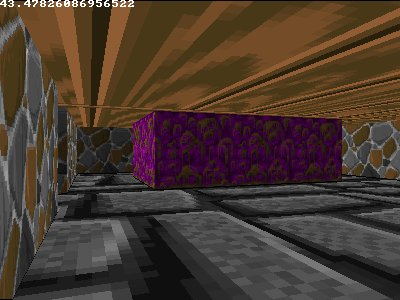So far, the whole level had the same floor texture everywhere. Since, in the way the level is described, all non-walls have code 0, this can't be used to give each square its own floortile texture. You could make non-wall tiles 0 or negative instead, then while raycasting a negative number means no wall, and the value can be used to say what floor texture has to be used there. If you want to do the same with the ceiling, you'd need another value for the ceiling textures too, so you could also consider using a separate map for the walls, floor and ceiling. Instead of doing that, here will now be demonstrated how to give each tile its own texture based on its coordinates: if the sum of its x and y coordinate on the map is even, it gets texture 3, if it's odd, it gets texture 4, this will give a checkerboard pattern.

To get the x and y coordinate of the current tile in the map, take the integer part of currentFloorX and currentFloorY. To get this, the for loop of the floor casting part is changed into this (the bold parts are new or changed):

 ``` //draw the floor from drawEnd to the bottom of the screen for(int y = drawEnd + 1; y < h; y++) { currentDist = h / (2.0 * y - h); //you could make a small lookup table for this instead double weight = (currentDist - distPlayer) / (distWall - distPlayer); double currentFloorX = weight * floorXWall + (1.0 - weight) * posX; double currentFloorY = weight * floorYWall + (1.0 - weight) * posY; int floorTexX, floorTexY; floorTexX = int(currentFloorX * texWidth) % texWidth; floorTexY = int(currentFloorY * texHeight) % texHeight; int checkerBoardPattern = (int(currentFloorX) + int(currentFloorY))) % 2; int floorTexture; if(checkerBoardPattern == 0) floorTexture = 3; else floorTexture = 4; //floor buffer[y][x] = (texture[floorTexture][texWidth * floorTexY + floorTexX] >> 1) & 8355711; //ceiling (symmetrical!) buffer[h - y][x] = texture[texWidth * floorTexY + floorTexX]; } }```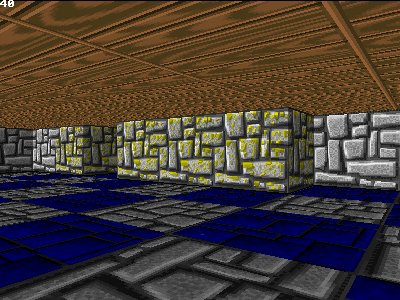In a similar way, it's also possible to choose the floor texture for each tile based on a map instead. The integer part of currentFloorX gives the coordinates of the current floortile in the map, while the fractional part gives the coordinate of the texel on the texture.

If you modify the checkerboard code from "(int(currentFloorX) + int(currentFloorY)) % 2" into "(int(currentFloorX + currentFloorY)) % 2", you don't get a checkerboard pattern but diagonal stripes instead, because now the fractional parts are added as well.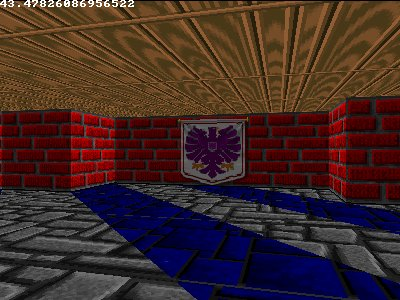## Vertical Version

As an alternative to the horizontal scanline based floor casting technique described above, it's also possible to work vertically. This allows to continue drawing the same vertical stripe a current wall stripe was drawn. However, this technique is slower because it requires perspective correct texture mapping, doing a division for every single pixel. In addition, the scanline based technique is also faster because scanline order is faster to render thanks to locality for memory caching.

The result looks the same (the floors are still horizontal), it's just rendered in a different way.

This technique works as follows: after you've drawn a vertical stripe from the wall, you do the floor casting for every pixel below the bottom wall pixel until the bottom of the screen. You need to know the exact coordinates of two points of the floor that are inside the current stripe, two such points that can easily be found are: the position of the player, and, the point of the floor right in front of the wall. Then, for every pixel, calculate the distance its projection on the floor has to the player. With that distance, you can find the exact location of the floor that pixel represents by using linear interpolation between the two points you found (the one at the wall and the one at your position).

Once you've done all the floor calculations, out of the exact position you can easily find the coordinates of the texel from the texture to get the color of the pixel you need to draw. Because the floor and ceiling are symmetrical, you know the texel coordinates of the ceiling texture are the same, you just draw it at the corresponding pixel in the upper half of the screen instead and can use a different texture for the ceiling and the floor.

The distance the projection of the current pixel is to the floor can be calculated as follows:

• If the pixel is in the center of the screen (in vertical direction), the distance is infinite.
• If the pixel is at the bottom of the screen, you can choose a certain distance, for example 1
• So all the pixels between those are between 1 and infinite, the distance the pixel represents in function of its height in the bottom half of the screen is inversely related as 1 / height. You can use the formula "currentDist = h / (2.0 * y - h)" for the distance of the current pixel.
• You can also precalculate a lookup table for this instead, since there are only h / 2 possible values (one half of the screen in vertical direction).
The linear interpolation, to get the exact floor location based on the current distance and the two known distances, can be done with a weight factor. This weight factor is "weight = (currentDist - distPlayer) / (distWall - distPlayer)", and since the current pixel will always be between the wall and the position of the player, the exact position is then: "currentFloorPos = weight * floorPosWall + (1.0 - weight) * playerPos". Note that distPlayer is actually 0, so the weight is actually "currentDist / distWall".

The code is not given in full this time, the floor casting is now done right after the wall casting, in the same x-loop. Don't forget to remove or disable the other floor casting code before adding this.

Right after the walls are drawn, the floor casting can begin. First the position of the floor right in front of the wall is calculated, and there are 4 different cases possible depending if a north, east, south or west side of a wall was hit. After this position and the distances are set, the for loop in the y direction that goes from the pixel below the wall until the bottom of the screen starts, it calculates the current distance, out of that the weight, out of that the exact position of the floor, and out of that the texel coordinate on the texture. With this info, both a floor and a ceiling pixel can be drawn. The floor is made darker.

 ``` for(int x = 0; x < w; x++) { //WALL CASTING // [SNIP... the floor casting code goes in the same x-for-loop as the wall casting, wall casting code not duplicated here] //FLOOR CASTING (vertical version, directly after drawing the vertical wall stripe for the current x) double floorXWall, floorYWall; //x, y position of the floor texel at the bottom of the wall //4 different wall directions possible if(side == 0 && rayDirX > 0) { floorXWall = mapX; floorYWall = mapY + wallX; } else if(side == 0 && rayDirX < 0) { floorXWall = mapX + 1.0; floorYWall = mapY + wallX; } else if(side == 1 && rayDirY > 0) { floorXWall = mapX + wallX; floorYWall = mapY; } else { floorXWall = mapX + wallX; floorYWall = mapY + 1.0; } double distWall, distPlayer, currentDist; distWall = perpWallDist; distPlayer = 0.0; if (drawEnd < 0) drawEnd = h; //becomes < 0 when the integer overflows //draw the floor from drawEnd to the bottom of the screen for(int y = drawEnd + 1; y < h; y++) { currentDist = h / (2.0 * y - h); //you could make a small lookup table for this instead double weight = (currentDist - distPlayer) / (distWall - distPlayer); double currentFloorX = weight * floorXWall + (1.0 - weight) * posX; double currentFloorY = weight * floorYWall + (1.0 - weight) * posY; int floorTexX, floorTexY; floorTexX = int(currentFloorX * texWidth) % texWidth; floorTexY = int(currentFloorY * texHeight) % texHeight; //floor buffer[y][x] = (texture[texWidth * floorTexY + floorTexX] >> 1) & 8355711; //ceiling (symmetrical!) buffer[h - y][x] = texture[texWidth * floorTexY + floorTexX]; } }```

## Next Part

Go directly to part III
Last edited: 2019